# Shannon information capacity

View legacy documentation

Photographic scientists and engineers stress the fact that no single number satisfactorily describes the ability of a photographic system to reproduce the small-scale attributes of the subject
 Shannon information capacity is now calculated from images of the Siemens star, with much better accuracy than slanted-edge measurements, which have been changed to give results similar to star measurements, but with less accuracy. Star measurements are the primary recommended method. The old slanted-edge method has been deprecated.

Nothing like a challenge! There is such a metric for electronic communication channels— one that specifies the maximum amount of information that can be transmitted through a channel. The metric includes the effects of sharpness and noise (grain in film). And a camera— or any digital imaging system— is such a channel.

The metric, first published in 1948 by Claude Shannon* of Bell Labs, has become the basis of the electronic communication industry. It is called the Shannon channel capacity or Shannon information transmission capacity C , and has a deceptively simple equation. (See the Wikipedia page on the Shannon-Hartley theorem for more detail.)

$$\displaystyle C = W \log_2 \left(1+\frac{S}{N}\right) = W \log_2 \left(\frac{S+N}{N}\right)$$

W is the channel bandwidth, which corresponds to image sharpness, S is the signal energy (the square of signal voltage), and N is the noise energy (the square of the RMS noise voltage), which corresponds to grain in film. It looks simple enough (only a little more complex than E = mc2 ), but the details must be handled with care. Fortunately you don’t need to know all the details to obtain good measurements. We present a few key points, then show how to calculate information capacity from images of the Siemens star. A secondary (less accurate) calculation, based on the slanted-edge, is at the bottom of this document. Technical details are in the green (“for geeks”) boxes.

*Claude Shannon was a genuine genius. The article, 10,000 Hours With Claude Shannon: How A Genius Thinks, Works, and Lives, is a great read.

Measurements of information capacity made with with slanted-edge images, where sharpness and noise are measured in separate locations, can be fooled by commonplace digital signal processing. Bilateral filtering, which combines noise reduction (lowpass filtering, i.e., smoothing) in areas that lack contrasty detail with sharpening (high frequency boost) in contrasty areas improves the measured signal-to-noise ratio, S/N, and hence increases measured information capacity C, but it removes fine, low contrast detail, i.e., it reduces the true information capacity.

We have mitigated this issue by using the Siemens star, where signal and noise are measured at the same location.

### Meaning of Shannon information capacity

In electronic communication channels the information capacity is the maximum amount of information that can pass through a channel without error, i.e., it is a measure of channel “goodness.” The actual amount of information depends on the code— how information is represented. But coding is not relevant to digital photography. What is important is the following hypothesis:

Hypothesis: Perceived image quality (assuming a well-tuned image processing pipeline), as well as the performance of machine vision and Artificial Intelligence (AI) systems, is proportional to information capacity, which is a function of both MTF (sharpness) and noise.

I stress that this statement is a hypothesis— a fancy mathematical term for a conjecture. It agrees with my experience, but it needs more testing (with a variety of images) before it can be accepted by the industry. Now that information capacity can be conveniently calculated with Imatest, we have an opportunity to learn more about it.

The information capacity, as we mentioned, is a function of both bandwidth W and signal-to-noise ratio, S/N. It’s important to use good measurements for both of these parameters.

In texts that introduce the Shannon capacity, bandwidth W is often assumed to be the half-power frequency, which is closely related to MTF50. Strictly speaking, this is only correct for white noise (which has a flat spectrum) and a simple low pass filter (LPF). But digital cameras have varying amounts of sharpening, and strong sharpening can result in response curves with large peaks that deviate substantially from simple LPF response. For this reason we prefer the integral form of the Shannon equation:

$$\displaystyle C = \int_0^W \log_2 \left( 1 + \frac{P(f)}{N(f)} \right) df = \int_0^W \log_2 \left(\frac{P(f)+N(f)}{N(f)} \right) df$$

As explained in the paper, “Measuring camera Shannon Information Capacity with a Siemens Star Image”, we have had to alter this equation to account for the two-dimensional nature of pixels.

$$\displaystyle C = 2 \pi\int_0^W \log_2 \left( 1 + \frac{P(f)}{N(f)} \right) f\: df = 2 \pi\int_0^W \log_2 \left(\frac{P(f)+N(f)}{N(f)} \right) f\: df$$

When we used slanted-edges, the choice of signal S presented serious issues when calculating the signal-to-noise ratio S/N because S can vary widely between images and even within an image. It is much larger in highly textured, detailed areas than it is in smooth areas like skies. A single value of S cannot represent all situations. This issue is handled well in the Siemens star, where the signal and noise power is calculated in each segment of the image (where a segment is a range of radii and angles).

### Siemens star method

A key challenge in measuring information capacity is how to define mean signal power S. Ideally, the definition should be based on a widely-used test chart. For convenience, the chart should be scale-invariant (so precise chart magnification does not need to be measured). And, as we indicated, signal and noise should be measured at the same location. For different observers to obtain the same result the chart design and contrast should be standardized.To that end we recommend a sinusoidal Siemens star chart similar to the chart specified in ISO 12233:2014/2017, Annex E. Contrast should be as close as possible to 50:1 (the minimum specified in the standard; close to the maximum achievable with matte media). Higher contrast can make the star image difficult to linearize. Lower contrast is acceptable, but should be reported with the results. The chart should have 144 cycles for high resolution systems, but 72 cycles is sufficient for low resolution systems. The center marker (quadrant pattern), used to center the image for analysis, should be 1/20 of the star diameter.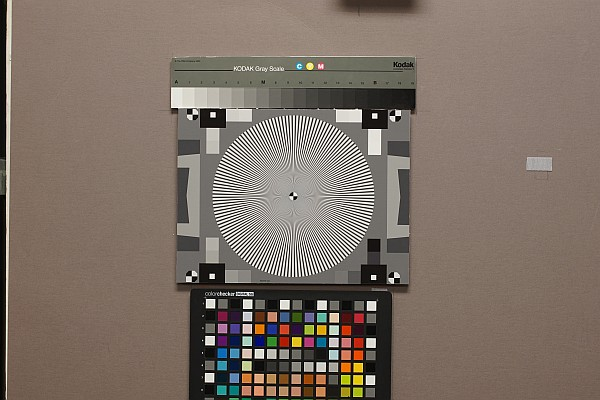Acquire a well-exposed image of the Siemens star in even, glare-free light. Exposures should be reasonably consistent when multiple cameras are tested. The mean pixel level of the linearized image inside the star should be in the range of 0.16 to 0.36. (The optimum has yet to be determined.)

The center of the star should be located as close as possible to the center of the image to minimize measurement errors caused by optical distortion (if present).
The size of the star in the image should be set so the maximum spatial frequency, corresponding to the minimum radius rmin, is larger than the Nyquist frequency fNyq, and, if possible, no larger than 1.3 fNyq, so sufficient lower frequencies are available for the channel capacity calculation. This means that a 144-cycle star with a 1/20 inner marker should have a diameter of 1400-1750 pixels and a 72-cycle star should have a diameter of 700-875 pixels. For high-quality inkjet printers, the physical diameter of the star should be at least 9 (preferably 12) inches (23 to 30 cm).

Other features may surround the chart, but the average background should be close to neutral gray (18% reflectance) to ensure a good exposure (it is OK to apply exposure compensation if needed). The figure on the right shows a typical star image in a 24-megapixel (4000×6000 pixel) camera.

Run the Star module, either in Rescharts (interactive; recommended for getting started) or as a fixed, batch-capable module (Star button on the left of the Imatest main window).

In the Star chart settings window, make sure the Calculate information capacity checkbox (near the bottom of the Settings section) is checked. The SNRI settings will be described later. If other settings are correct, press OK.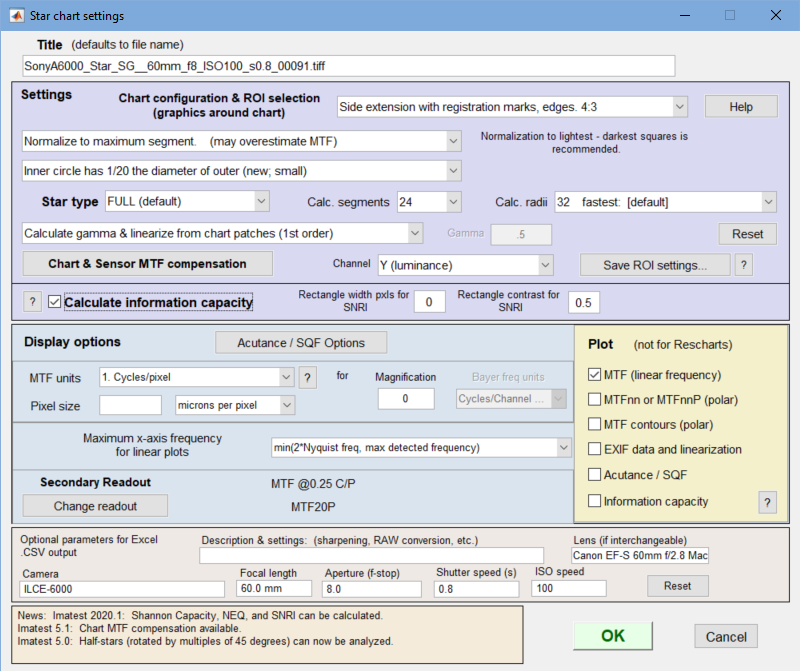Star settings window

When OK is pressed the image will be analyzed. Any of several displays can be selected in Rescharts. The table below shows displays that are only available for information capacity measurements.

 Main display Secondary display Description 9.  Information capacity, SNRI SNR (ratio) Signal-to-Noise Ratio (S/N) as a function of spatial frequency for the mean segment and up to 8 individual segments SNR (dB) SNR (dB) as a function of frequency for the mean segment, etc. Signal, Noise Signal, noise, and (S+N)/N (dB) as a function of frequency for the mean segment. Signal, 10X Noise Signal, 10X noise, and (S+N)/N (dB) as a function of frequency for the mean segment. Useful for visualizing low levels of noise NEQ Noise Equivalent Quanta as a function of frequency 10. Input-noiseless Diff, etc. Noise-only (input-noiseless) Display noise-only (with signal removed). This is a remarkable result — possibly the first time that noise has been measured and visualized in the presence of a signal. Loss (input-ideal) Input − Lossless (test chart image). Shows data that has been attenuated. Difficult to interpret. Input image Input image (unmodified) Noiseless image Ideal (noiseless) input image (with MTF loss), derived from Sideal. Ideal image (no MTF loss) “Ideal” image with no MTF loss (represents the original test chart). Noise-only (linear) Noise-only linearized. Typically darker than the gamma-encoded version. Input image (linear) Input image linearized. Typically darker than the gamma-encoded version.

Two Rescharts displays are specifically designed for information capacity results: 9. Information capacity, SNRI, and 10. Input-noiseless Diff, etc.  Here is a result from Star run in Rescharts for a raw image (converted to TIFF with dcraw using the 24-bit sRGB preset; gamma ≅ 2.2) for a high quality 24-megapixel APS-C camera.

### Information capacity plot

The plot below shows signal, noise, and (Signal+Noise)/Noise (db)  for the 24-megapixel APS-C Sony A6000, set at ISO 400.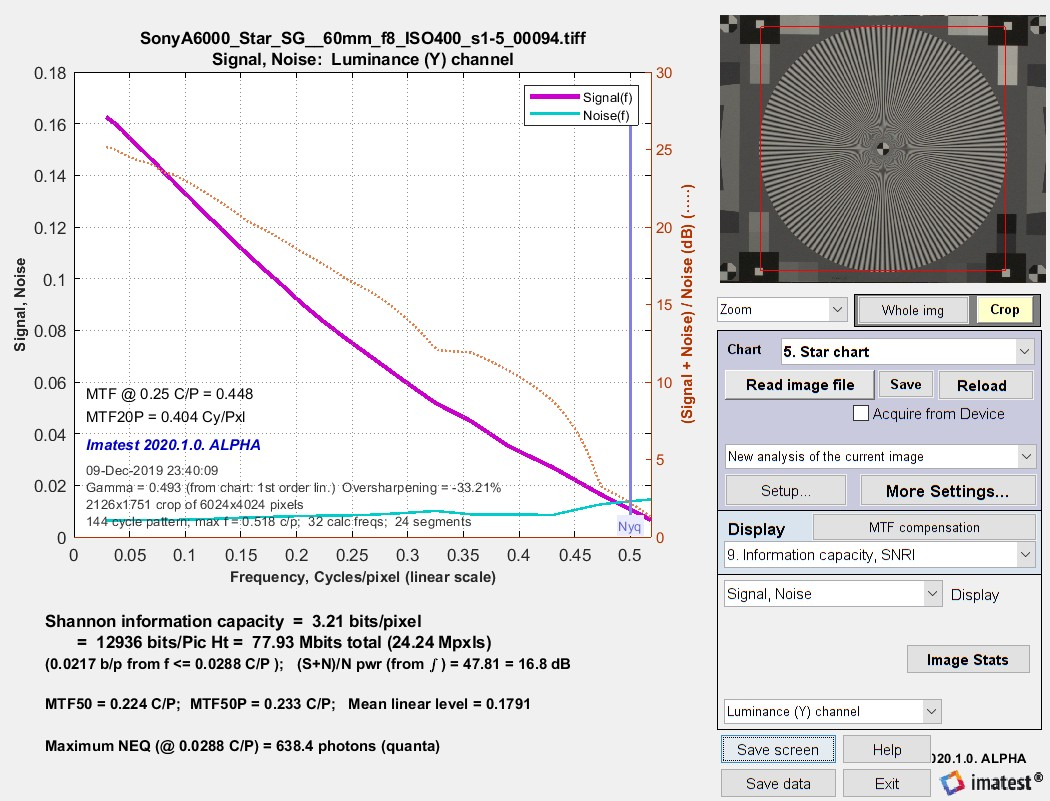Signal, Noise, and Shannon information capacity (3.21 bits/pixel) from a
raw image (converted to TIFF) from a high-quality 24-megapixel APS-C camera @ ISO 400.

 This shows results for an in-camera JPEG the same image capture. The curve has a “bump” that is characteristic of sharpening. Note that the Shannon information capacity is lower than the raw image, even though the JPEG is sharpened. This is happening because the high frequency noise is boosted, along with the signal.   Signal, Noise, and Shannon information capacity (2.92 bits/pixel) from an in-camera JPEG image from a high-quality 24-megapixel APS-C camera @ ISO 400.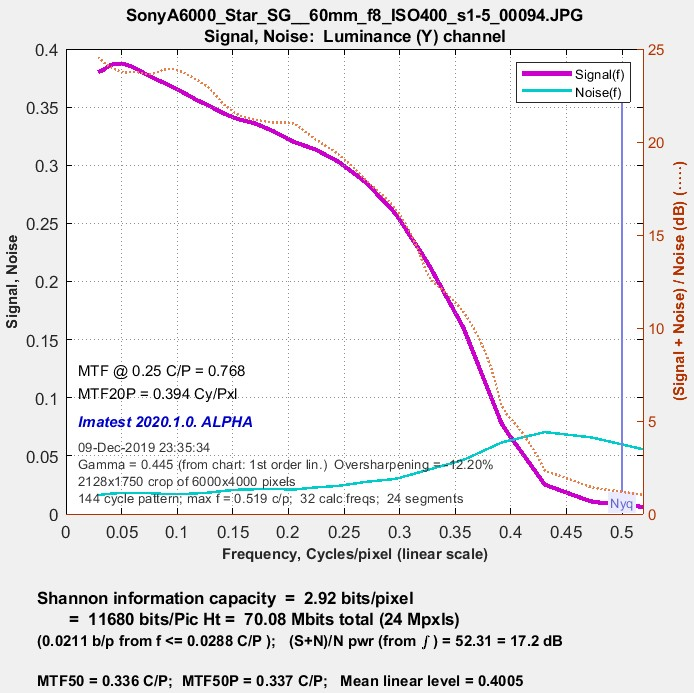### Image plot (Input-noiseless Diff, etc.)

The noise-only (input-noiseless difference) plot is of particular interest because images that allow measurement and visualization of noise measured in the presence of a signal (with the sinusoldal star pattern removed) have not been previously available. Because noise is very low, and hence hard to see, at ISO 400, we illustrate noise at ISO 25600 (the maximum for the APS-C camera) for both TIFF from raw and JPEG images. The  Copy image  button on the right copies the image to the clipboard, where it can be pasted into an image editor/viewer or the Image Statistics module for further analysis.Noiseless image for APS-C camera, raw/TIFF image, ISO 25600.

 The image on the right is an in-camera JPEG from the same capture at the above image (ISO 25600). It looks very different from the raw/TIFF image because noise reduction is present. The images below are for raw/TIFF and in-camera JPEG images from the same camera acquired at ISO 400.
Green is for geeks. Do you get excited by a good equation? Were you passionate about your college math classes? Then you’re probably a math geek a member of a misunderstood but highly elite fellowship. The text in green is for you. If you’re normal or mathematically challenged, you may skip these sections. You’ll never know what you missed.

Calculating Shannon capacity with Siemens star images

The pixel levels of most interchangeable images (typically encoded in color spaces such as sRGB or Adobe RGB) are gamma-encoded. For these files, pixel level ≅ (sensor illumination)1/gamma, where gamma (typically around 2.2) is the the intended viewing gamma for the color space (display brightness = (pixel level)gamma). To analyze these files they must be linearized by raising the pixel level to the gamma power. RAW files usually don’t need to be linearized (if they were demosaiced without gamma-encoding, i.e., gamma = 1).

The image of the ntotal cycle Siemens star is divided into nr = 32 or 64 radial segments and ns =8 (recommended), 16, or 24 angular segments. Each segment has a period (angular length in radians) P = 2πntotal/ns and contains nk =ntotal / nscycles and kn signal points, each at a known angular location φ, in the range {0, P}.

We assume that the ideal signal in the segment has the form

$$\displaystyle S_{ideal}(\phi) = \sum_{j=1}^{2} a_j \cos \bigl(\frac{2 \pi j n_k \phi}{P} \bigr) + b_j \sin \bigl(\frac{2 \pi j n_k \phi}{P} \bigr)$$

a and b are calculated using the Fourier series coefficient equations, derived from the Wikipedia Fourier Series page, Equation 1.

$$\displaystyle a_j = \frac{2}{P}\int_P S(\phi) \cos \bigl(\frac{2 \pi j n_k \phi}{P} \bigr) d\phi;\quad b_j = \frac{2}{P}\int_P S(\phi) \sin \bigl(\frac{2 \pi j n_k \phi}{P} \bigr) d\phi$$

where S(φ) is the measured signal (actually, signal + noise) in the segment. [Note that although this equation is not in the ISO 12233:2017 standard, it fully satisfies the intent of Appendix F, Step 5 (“A sine curve with the expected frequency is fitted into the measured values by minimizing the square error.”)]

Noise is  $$\displaystyle N(\phi) = S(\phi)-S_{ideal}(\phi)$$

The frequency f in Cycles/Pixel of a segment centered at radius r (in pixels) is $$\displaystyle f = \frac{n_{total}}{2 \pi r}$$.  An interesting consequence of this equation is that it’s easy to locate the Nyquist frequency (0.5 C/P):  $$\displaystyle r = \frac{n_{total}}{\pi}$$ = 45.8 pixels for ntotal = 144 cycles.

A small adjustment (not described here) is made in case f is slightly different from the expected value due to centering errors, optical distortion, or other factors.

Signal power is $$\displaystyle P(f) = \sigma^2(S_{ideal}(f))$$. Noise power is $$\displaystyle N(f) = \sigma^2(N)$$, where σ2 is variance (the square of standard deviation). Note that signal + noise power is $$\displaystyle P(f)+N(f) = \sigma^2(S)$$. [Note: From the context of Shannon: “Communication in the presence of noise”, we assume that N(f) is the noise measured in the presence of signal Sideal(f); not narrow-band noise of frequency f.]

The full one-dimensional equation for Shannon capacity was presented in Shannon’s second paper in information theory, “Communication in the Presence of Noise,” Proc. IRE, vol. 37, pp. 10-21, Jan. 1949, Eq. (32). This equation cannot be used directly because the pixels under consideration are two-dimensional.

$$\displaystyle C = \int_0^W \log_2 \left( 1 + \frac{P(f)}{N(f)} \right) df = \int_0^W \log_2 \left(\frac{P(f)+N(f)}{N(f)} \right) df$$     [One-dimensional; not used]

This equation has to be converted into two dimensions since pixels (here) have units of area. (They have units of distance for linear measurements like MTF.)

$$\displaystyle C = \int \int_0^W \log_2 \left(\frac{P(f_x,f_y)+N(f_x,f_y)}{N(f_x,f_y)} \right) df_x df_y$$

where f_x and f_y are frequencies in the x and y-directions, respectively. In order to evaluate this integral, we translate x and y into polar coordinates, r and θ.

$$\displaystyle C = \int_0^{2 \pi} \int_0^W \log_2 \left(\frac{P(f_r,f_θ)+N(f_r,f_θ)}{N(f_r,f_θ)} \right) f_r \: df_r df_θ$$

Since S and N are only weakly dependent on θ, we can rewrite this equation in one-dimension.

$$\displaystyle C = 2 \pi \int_0^W \log_2 \left(\frac{P(f)+N(f)}{N(f)} \right) f \: df$$

Very geeky: The limiting case for Shannon capacity. Suppose you have an 8-bit pixel. This corresponds to 256 levels (0-255). If you consider the distance of 1 between levels to be the “noise”, then the S/N part of the Shannon equation is log2(1+2562) ≅ 16. The maximum bandwidth where information can be transmitted correctly W— the Nyquist frequency— is 0.5 cycles per pixel. (All signal energy above Nyquist is garbage— disinformation, so to speak.) So C = W log2(1+(S/N)2) = 8 bits per pixel, which is where we started. Sometimes it’s comforting to travel in circles.

### Summary

• Shannon capacity C has long been used as a measure of the goodness of electronic communication channels.
• We hypothesize that C can be used as a figure of merit for evaluating camera quality, especially for machine vision cameras. (Consumer cameras have to be carefully tuned to reach their potential, i.e., to make pleasing images). It provides a fair basis for comparing cameras, especially when used with images converted from raw with minimal processing.
• Imatest calculates the Shannon capacity C for the Y (luminance; 0.212*R + 0.716*G + 0.072*B) channel of digital images, which approximates the eye’s sensitivity.
• Shannon capacity has not been used to characterize photographic images because it was difficult to calculate and interpret. But now it can be calculated easily, its relationship to photographic image quality is open for study.
• We stress that C is still an experimental metric for image quality. Much work needs to be done to demonstrate its validity. Noise reduction and sharpening can distort its measurement. Imatest results for C should therefore be regarded with a degree of skepticism; they should not be accepted uncritically as “the truth.”

### Further considerations and calculations

• C is ordinarily measured in bits per pixel. The total capacity is $$C_{total} = C \times \text{number of pixels}$$.
• The channel must be linearized before C is calculated, i.e., an appropriate gamma correction (signal = pixel levelgamma, where gamma ~= 2) must be applied to obtain correct values of S and N. The value of gamma (close to 2) is determined from runs of any of the Imatest modules that analyze grayscale step charts: Stepchart, Colorcheck., Multicharts, Multitest, SFRplus, or eSFR ISO.
• Digital cameras apply varying degrees of noise reduction, which may make an image look “prettier,” but removes low contrast signals at high spatial frequencies (which represent real texture information). When slanted-edges are used for measuring C, noise reduction makes the Shannon capacity appear better than it really is, but it results in a loss of information— especially in low contrast textures— resulting in images where textures look “plasticy” or “waxy.” The exact amount of noise reduction cannot be determined with a simple slanted-edge target (especially with JPEG images from cameras). The Log Frequency-Contrast chart and module provides some information on noise reduction vs. image contrast, but is not easy to apply to the Shannon capacity calculation. Noise reduction results in an unusually rapid dropoff the noise spectrum— which is evident when several cameras are compared (demosaicing alone typically cause the noise spectrum to drop by half at the Nyquist freqnency). For all these reasons we recommend working with raw images when possible.

#### Slanted-edge results

Because the old (pre-2020.1) slanted-edge calculation is no longer recommended for Shannon capacity, text below is shown in gray.

Shannon capacity measured from slanted-edges is NOT a trustworthy metric for JPEG files from most consumer cameras because image processing varies over the image surface and noise reduction improves measured Signal-to-Noise Ratio in smooth regions while removing information. It is much more reliable when measured from Star charts, where signal and noise is measured in the same location.

In the original slanted-edge calculation we start with a standard value of signal, Sstd: the difference between the white and black zones in a reflective surface such as the ISO 12233 test chart. This represents a tonal range of roughly 80:1 (a pixel ratio of about 9:1 for for an image encoded with gamma = 1/2: typical for a wide range of digital cameras). Then we plot Shannon capacity C for a range of S from 0.01*Sstd (representing very low contrast regions) to 2*Sstd (about a 160:1 contrast range, which represents an average sunny day scene— fairly contrasty). Imatest displays values of C for three contrast levels relative to Sstd: 100% (representing a contrasty scene), 10% (representing a low contrast scene), and 1% (representing smooth areas). Results are shown below.

The Signal S, which is a part of the equation for Shannon capacity C, varies from image to image and even within images. It is large for detailed, textured areas and small for smooth areas like skies. Sharpness (i.e., bandwidth W) dominates image quality in detailed areas where S is large; noise N is more important in smooth areas where S is small.For this reason we calculate C for several values of S. The 100% contrast value is for Sstd, the difference between white and black reflective surfaces. C is also calculated for contrasts of 10% and 1% of Sstd, representing low contrast images and smooth areas, respectively.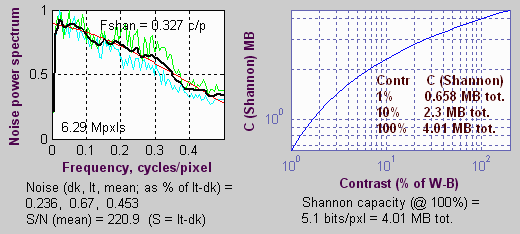Imatest displays noise and Shannon capacity plots at the bottom of the Chromatic aberration figure if the (Plot) Shannon capacity and Noise spectrum (in CA plot) checkbox in the SFR input dialog box is checked (the default is unchecked) and the selected region is sufficiently large. Here is a sample for the Canon EOS-10D.

The noise spectrum plot is experimental. Its rolloff is strongly affected by the amount of noise reduction. The pale green and cyan lines represent two different calculation methods. The thick black line is the average of the two. The red line is a second order fit. Noise spectrum will become more meaningful as different cameras are compared.

RMS noise voltage in the dark and light areas is expressed as a percentage of the difference between the light and dark signal levels, i.e., the standard signal S = Sstd., i.e., noise is actually N/Sstd. The inverse of mean (the average of the two) is used as S/N in the equation for C.

$$C = W \log_2 \bigl( (S/N)^2 +1 \bigr) = 3.322\; W \log_{10}\bigl( (S/N)^2 + 1 \bigr)$$

Shannon capacity C is calculated and displayed for three contrast levels.

Contrast Signal S Description
100% The standard signal, S = Sstd This is about an 80:1 contrast ratio— a moderately contrasty image.
Indicates image quality for contrasty images.
Weighs sharpness more heavily than noise.
10% S = Sstd /10 Indicates image quality for low contrast images.
1% S = Sstd /100 This represents an extremely low contrast image.
Indicates image quality in smooth areas such as skies.
Weighs noise more heavily.

The values of C are meaningful only in a relative sense— only when they are compared to a range of other cameras. Here are some typical results, derived from ISO 12233 charts published on the internet.

Camera Pixels V x H
(total Mpixels)
MTF50
LW/PH
MTF50C
LW/PH
S/N ISO C (MB)
100%
C (MB)
10%
C (MB)
1%
Canon EOS-10D 2048×3072 (6.3) 1325 1341 221 100 4.01 2.30 0.66
Canon EOS-1Ds 2704×4064 (11) 1447 1880 184 100 7.18 4.01 1.02 Little built-in sharpening.
Kodak DCS-14n 3000×4500 (13.5) 2102 2207 272 100? 10.0 5.92 1.90 No anti-aliasing filter.
Strong noise reduction.
Nikon D100 2000×3008 (6) 1224 1264 148 200? 3.43 1.85 0.40
Nikon D70 2000×3008 (6) 1749 1692 139 ? 4.53 2.42 0.50 Strikingly different response
from D100. Less aggressive anti-aliasing.
Sigma SD10 1512×2268 (3.4) 1363 1381 288 100 3.20 1.9 0.63 Foveon sensor. No anti-aliasing
filter. Very high MTF50C and response at Nyquist.
Canon G5 1944×2592 (5) 1451 1361 94 ? 2.89 1.43 0.20 Strongly oversharpened.
Sony DSC-F828 2448×3264 (8) 1688 1618 134 64 4.67 2.47 0.49 Compact 8 MP digital with excellent lens. S/N and C are expected to degrade at high ISO.

Performance measurements were taken from the edge located about 16% above the center of the image.

Here are some additional examples, illustrating unusual noise spectra. The Kodak DCS-14n shows a steep rolloff indicative of extreme noise reduction. This is reflected in the unusually high Shannon capacity at 1% contrast.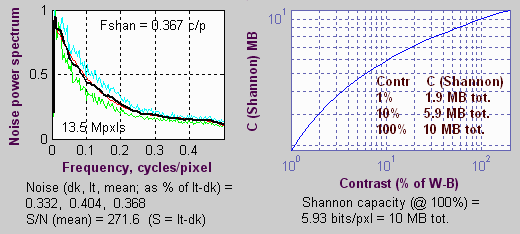The Olympus E-1 has an unusual noise spectrum, with a spike at Nyquist. I don’t know what to make of it.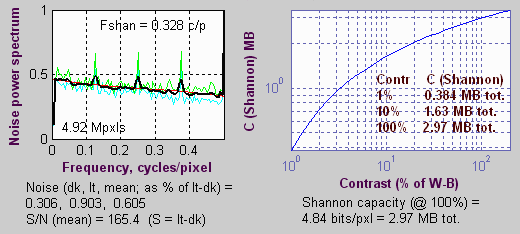#### Summary for slanted-edge measurements

• Shannon capacity C has long been used as a measure of the goodness of electronic communication channels.
• Imatest calculates the Shannon capacity C for the Y (luminance; 0.212*R + 0.716*G + 0.072*B) channel of digital images, which approximates the eye’s sensitivity.
• The calculation of C is an approximation: it is not precise, but may be useful for comparing the performance of digital cameras (or scanned film images).
• We hypothesize that C is closely related to overall image quality; that it provides a fair basis for comparing cameras with different pixel counts, sharpening, and noise levels.
• Because of non-uniform signal processing, C calculated from camera JPEG images in not trustworthy. It is better with raw images.
• We display values of C that correspond to three signal levels, 100%, 10% and 1%, representing moderately contrasty images, low contrast images, and smooth areas.
• Shannon capacity has not been used to characterize photographic images because it was difficult to calculate and interpret. But now it can be calculated easily, its relationship to photographic image quality is open for study.
• We stress that C is still an experimental metric for image quality. Much work needs to be done to demonstrate its validity. Noise reduction and sharpening can distort its measurement. Imatest results for C should therefore be regarded with a degree of skepticism; they should not be accepted uncritically as “the truth.”

#### Further considerations and calculations

• Since Imatest displays S and N as voltage rather than power or energy (both of which are proportional to the square of voltage), the equation used to evaluate Shannon capacity per pixel is $$C_P = W \log_2 \bigl( (S/N)^2 + 1 \bigr)$$, where W is measured in cycles per pixel. The total capacity is $$C = C_P \times \text{number of pixels}$$.
• The channel must be linearized before C is calculated, i.e., an appropriate gamma correction (signal = pixel levelgamma, where gamma ~= 2) must be applied to obtain correct values of S and N. The value of gamma (close to 2) is determined from runs of any of the Imatest modules that analyze grayscale step charts: Stepchart, Colorcheck., Multicharts, Multitest, SFRplus, or eSFR ISO.
• Digital cameras apply varying degrees of noise reduction, which may make an image look “prettier,” but removes low contrast signals at high spatial frequencies (which represent real texture information). When slanted-edges are used for measuring C, noise reduction makes the Shannon capacity appear better than it really is, but it results in a loss of information— especially in low contrast textures— resulting in images where textures look “plasticy” or “waxy.” The exact amount of noise reduction cannot be determined with a simple slanted-edge target (especially with JPEG images from cameras). The Log Frequency-Contrast chart and module provides some information on noise reduction vs. image contrast, but is not easy to apply to the Shannon capacity calculation. Noise reduction results in an unusually rapid dropoff the noise spectrum— which is evident when several cameras are compared (demosaicing alone typically cause the noise spectrum to drop by half at the Nyquist frequency). For all these reasons we recommend working with raw images when possible.

Because of a number of factors (noise reduction, the use of MTF50C to approximate W, the arbitrary nature of S, etc.) the Shannon capacity calculated from slanted-edges is an approximation. But it can be useful for comparing different cameras.

 Calculating Shannon capacity with slanted-edges The measurement of Shannon capacity is complicated by two factors. The voltage in the image sensor is proportional to the energy (the number of photons) striking it. Since Shannon’s equations apply to electrical signals, I’ve stuck to that domain.   The pixel level of standard digital image files is proportional to the sensor voltage raised to approximately the 1/2 power. This is the gamma encoding, designed to produce a pleasing image when the luminance of an output device is proportional to the pixel level raised to a power of 2.2 (1.8 for Macintosh). This exponent is called the gamma of the device or the image file designed to work with the device. Gamma = 2.2 for the widely-used sRBG and Adobe RGB (1998) color spaces. Since I need to linearize the file (by raising the pixel levels to a power of 2) to obtain a correct MTF calculation, I use the linearized values for calculating Shannon capacity, C. The correct, detailed equation for Shannon capacity was presented in Shannon’s second paper in information theory, “Communication in the Presence of Noise,” Proc. IRE, vol. 37, pp. 10-21, Jan. 1949. $$\displaystyle C_1 = \int_0^W \log \bigl( 1 + \frac{P(f)}{N(f)} \bigr) df$$ W is maximum bandwidth, P(f) is the signal power spectrum (the square of the MTF) andN( f ) is the noise power spectrum. There are a number of difficulties in evaluating this integral. Because P and N are calculated by different means, they are scaled differently. P(f) is derived from the Fourier transform of the derivative of the edge signal, while N( f ) is derived from the Fourier transform of the signal itself. And noise reduction removes information while reducing N( f ) at high spatial frequencies below its correct value. For this reason, until we solve the scaling issues we use the simpler, less accurate, but less error-prone approximation, $$C = W \log_2 \bigl( (S/N)^2 + 1 \bigr)$$ where bandwidth W is traditionally defined as the channel’s -3 dB (half-power) frequency, which corresponds to MTF50,S is standard (white - black) signal voltage, and N is RMS noise voltage. The square term converts voltage into power. S/N (the voltage signal-to-noise ratio) is displayed by Imatest. (S/N can refer to voltage or power in the literature; you have to read carefully to keep it straight.) Strictly speaking, this approximation only holds for white noise and a fairly simple (usually second-order) rolloff. It holds poorly when P( f ) has large response peaks, as it does in oversharpened digital cameras. The standardized sharpening algorithm comes to the rescue here. Imatest uses MTF50C (the 50% MTF frequency with standardized sharpening) to approximate W. This assures that P( f ) rolls off in a relatively consistent manner in different cameras: it is an excellent relative indicator of the effective bandwidth W. RMS (root mean square) noise voltage N is the standard deviation (sigma) of the linearized signal in either smooth image area, away from the edge. It is relatively easy to measure using the slanted edge pattern because the dynamic range of digital cameras is sufficient to keep the levels for the white and black regions well away from the limiting values (pixel levels 0 and 255). Typical average (mean) pixel values are roughly 18-24 for the dark region and 180-220 for the light region, depending on exposure. Imatest uses the average of the noise in the two regions to calculate Shannon capacity. It displays noise as N/S: normalized to (divided by) the difference between mean linearized signal level of the white and black regions, S. Noise power N doesn’t tell the whole story of image quality. Noise spectral density plays an important role. The eye is more sensitive to low frequency noise, corresponding to large grain clumps, than to high frequency noise. To determine the precise effect of grain, you need to include its spectral density, the degree of enlargement, the viewing distance, and the MTF response of the human eye. High frequency noise that is invisible in small enlargements may be quite visible in big enlargements. Noise metrics such as Kodak’s print grain index, which is perceptual and relative, takes this into account. Fortunately the noise spectrum of digital cameras varies a lot less than film. It tends to have a gradual rolloff (unless significant noise reduction is applied), and remains fairly strong at the Nyquist frequency. It’s not a major factor in comparing cameras— the RMS noise level is far more important. Very geeky: The limiting case for Shannon capacity. Suppose you have an 8-bit pixel. This corresponds to 256 levels (0-255). If you consider the distance of 1 between levels to be the “noise”, then the S/N part of the Shannon equation is log2(1+2562) ≅ 16. The maximum bandwidth where information can be transmitted correctly W— the Nyquist frequency— is 0.5 cycles per pixel. (All signal energy above Nyquist is garbage— disinformation, so to speak.) So C = W log2(1+(S/N)2) = 8 bits per pixel, which is where we started. Sometimes it’s comforting to travel in circles.

History

R. Shaw, “The Application of Fourier Techniques and Information Theory to the Assessment of Photographic Image Quality,” Photographic Science and Engineering, Vol. 6, No. 5, Sept.-Oct. 1962, pp.281-286. Reprinted in “Selected Readings in Image Evaluation,” edited by Rodney Shaw, SPSE (now SPIE), 1976.